# Test: Lines And Angles- 1

## 25 Questions MCQ Test NCERT Mathematics for CAT Preparation | Test: Lines And Angles- 1

Description
Attempt Test: Lines And Angles- 1 | 25 questions in 25 minutes | Mock test for Class 9 preparation | Free important questions MCQ to study NCERT Mathematics for CAT Preparation for Class 9 Exam | Download free PDF with solutions
QUESTION: 1

### If the supplement of an angle is three times its complement, then angle is:

Solution:

Let the ∠x be the required angle
Thus, according to the question
180−x=3(90−x)
=>180−x=270−3x
=>2x=90
=>x=45

QUESTION: 2

Solution:
QUESTION: 3

### The angle between the bisectors of two adjacent supplementary angles is :

Solution: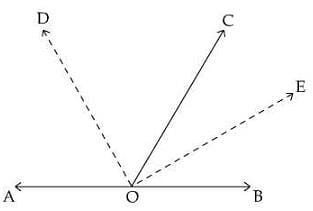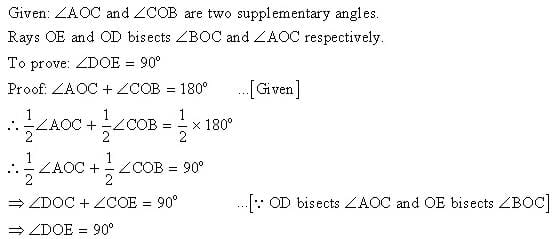QUESTION: 4

Which of the following is true?
(i) A triangle can have two obtuse angles.
(ii) A triangle can have all angles equal to 60°.
(iii) A triangle can have all angles more than 60°.

Solution:
QUESTION: 5

If two parallel lines are cut by a transversal, then the pairs of ___________ angles are congruent.

Solution:

If two parallel lines are cut by a transversal, the corresponding angles are congruent. If two lines are cut by a transversal and the corresponding angles are congruent, the lines are parallel.

QUESTION: 6

If two angles are complementary of each other, then each angle is :

Solution:
QUESTION: 7

X lies in the interior of ∠BAC. If ∠BAC = 70° and ∠BAX = 42° then ∠XAC =?

Solution:
QUESTION: 8

Which of the following statements is false?

Solution:

The correct option is Option B.

Infinite number of lines can pass through one point.

QUESTION: 9

An angle is 14° more than its complementary angle, then angle is :

Solution:

Let the angle be x
Then the complement angle will be 90 −x

Given x is 14 more than 90 −x
⟹ x = 14 + 90 −x
⟹ 2x = 104
⟹ x = 52
The measure of the angle is 52

QUESTION: 10

In the given figure, straight lines PQ and RS intersect at O. If the magnitude of θ is 3 times that of ψ, then (∠φ) is equal to :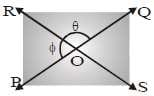Solution:
QUESTION: 11

Two parallel lines have:

Solution:
QUESTION: 12

How many degrees are there in an angle which equals one-fifth of its supplement?

Solution:
QUESTION: 13

Two angles whose measures are a & b are such that 2a - 3b = 60° then 5b = ?, if they form a linear pair:

Solution:
QUESTION: 14

If two parallel lines are intersected by a transversal then the bisectors of the interior angles form a :

Solution:
if two parallel lines are intersected by a transversal then prove that bisectors of the interior angles form a triangle.if two parallel lines are intersected by a transversal then prove that bisectors of the interior angles form a rectangle.

QUESTION: 15

If one angle of triangle is equal to the sum of the other two angles then triangle is :

Solution: In right angle triangle one angle in 90 degree, so the sum of other two angles will be 90 degree as per angle sum property.
QUESTION: 16

If the arms of one angle are respectively parallel to the arms of another angle, then the two angles are :

Solution:
QUESTION: 17

Which one of the following is not correct?

Solution:
QUESTION: 18

If l is any given line and P is any point not lying on l, then the number of parallel lines that can be drawn through P, parallel to l would be :

Solution:

Draw a line l and a point P not lying on l.

Now, we can draw a straight line parallel to l which passes through P.

Clearly from the figure, only one line can be drawn which is parallel to l and passes through P.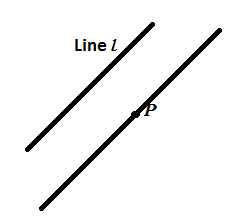QUESTION: 19

Which one of the following statements is not false?

Solution:
QUESTION: 20

There are four lines in a plane no two of which are parallel. The maximum number of points in which they can intersect is :

Solution:
QUESTION: 21

An exterior angle of a triangle is 80° and two interior opposite angles are equal. Measure of each of these angles is :

Solution:
QUESTION: 22

The angle which is equal to 8 times its complement is :

Solution: Complement angle of 80 degree is 10 degree (90-80), which gives 80 degree when multlipied by 8.
QUESTION: 23

If two supplementary angles are in the4 ratio 2 : 7, then the angles are :

Solution: Let, One angle be 2x and another angle be 7x. Now, 2x+7x=180 9x =180 x =180/9=20 Therefore, one angle=2*20=40 Another angle=7*20=140
QUESTION: 24

In the given figure, the value of x which makes POQ a straight line is :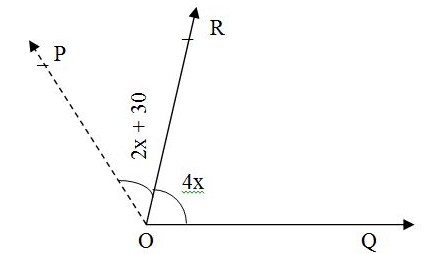Solution:

For POQ=straight line →∠ POQ=180o

2x+30+4x=180

6x+30=180

6x=150

x=25o.

QUESTION: 25

If two interior angles on the same side of a transversal intersecting two parallel lines are in the ratio 2 : 3, then the largest of two angles is :

Solution:Use Code STAYHOME200 and get INR 200 additional OFF Use Coupon Code